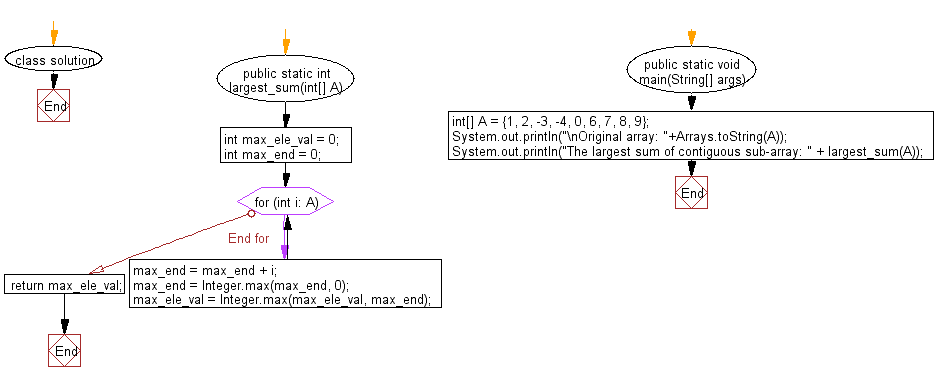﻿ Java: Subarray within an array which has the largest sum# Java Array Exercises: Find contiguous subarray within a given array of integers which has the largest sum

## Java Array: Exercise-66 with Solution

Write a Java program to find contiguous subarray within a given array of integers which has the largest sum.

In computer science, the maximum sum subarray problem is the task of finding a contiguous subarray with the largest sum, within a given one-dimensional array A[1...n] of numbers. Formally, the task is to find indices and with, such that the sum is as large as possible.

Example:
Input :
int[] A = {1, 2, -3, -4, 0, 6, 7, 8, 9}
Output:
The largest sum of contiguous sub-array: 30

Sample Solution:

Java Code:

``````import java.util.Arrays;
class solution
{
public static int largest_sum(int[] A)
{
int max_ele_val = 0;
int max_end = 0;
for (int i: A)
{
max_end = max_end + i;
max_end = Integer.max(max_end, 0);

max_ele_val = Integer.max(max_ele_val, max_end);
}
return max_ele_val;
}
public static void main(String[] args)
{
int[] A = {1, 2, -3, -4, 0, 6, 7, 8, 9};
System.out.println("\nOriginal array: "+Arrays.toString(A));
System.out.println("The largest sum of contiguous sub-array: " + largest_sum(A));
}
}
```
```

Sample Output:

```Original array: [1, 2, -3, -4, 0, 6, 7, 8, 9]
The largest sum of contiguous sub-array: 30
```

Flowchart:Java Code Editor:

Improve this sample solution and post your code through Disqus

What is the difficulty level of this exercise?

Test your Programming skills with w3resource's quiz.

﻿

## Java: Tips of the Day

countOccurrences

Counts the occurrences of a value in an array.

Use Arrays.stream().filter().count() to count total number of values that equals the specified value.

```public static long countOccurrences(int[] numbers, int value) {
return Arrays.stream(numbers)
.filter(number -> number == value)
.count();
}
```

Ref: https://bit.ly/3kCAgLb